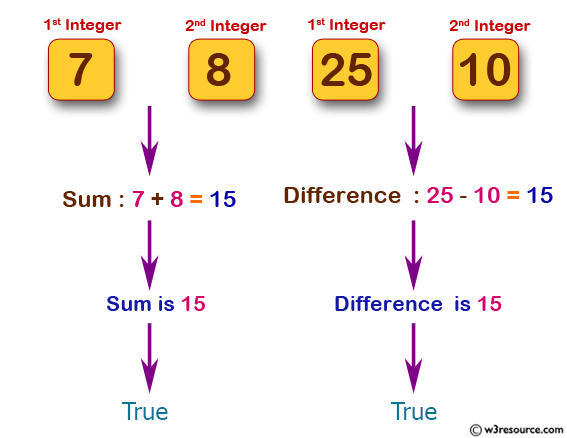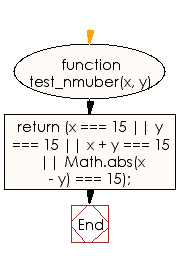# JavaScript: Check two given integer values and return true if one of the number is 15 or if their sum or difference is 15

## JavaScript Basic: Exercise-45 with Solution

Write a JavaScript program to check two given integer values and return true if one of the number is 15 or if their sum or difference is 15.

Pictorial Presentation:Sample Solution:

HTML Code:

``````<!DOCTYPE html>
<html>
<meta charset="utf-8">
<meta name="viewport" content="width=device-width">
<title>JavaScript program to check two given integer values and return true if one of the number is 15 or if their sum or difference is 15.</title>
<body>

</body>
</html>
```
```

JavaScript Code:

``````function test_number(x, y) {
return (x === 15 || y === 15 || x + y === 15 || Math.abs(x - y) === 15);
}

console.log(test_number(15, 9));
console.log(test_number(25, 15));
console.log(test_number(7, 8));
console.log(test_number(25, 10));
console.log(test_number(5, 9));
console.log(test_number(7, 9));
console.log(test_number(9, 25));
```
```

Sample Output:

```true
true
true
true
false
false
false
```

Flowchart:ES6 Version:

``````function test_number(x, y) {
return (x === 15 || y === 15 || x + y === 15 || Math.abs(x - y) === 15);
}

console.log(test_number(15, 9));
console.log(test_number(25, 15));
console.log(test_number(7, 8));
console.log(test_number(25, 10));
console.log(test_number(5, 9));
console.log(test_number(7, 9));
console.log(test_number(9, 25));
``````

Live Demo:

Improve this sample solution and post your code through Disqus

What is the difficulty level of this exercise?

Test your Programming skills with w3resource's quiz.

﻿

## JavaScript: Tips of the Day

Returns the sum of the powers of all the numbers from start to end (both inclusive)

Example:

```const sumPower = (end, power = 2, start = 1) =>
Array(end + 1 - start)
.fill(0)
.map((x, i) => (i + start) ** power)
.reduce((a, b) => a + b, 0);
console.log(sumPower(10)); // 385
console.log(sumPower(10, 3)); // 3025
console.log(sumPower(10, 3, 5)); // 2925
```

Output:

```385
3025
2925
```